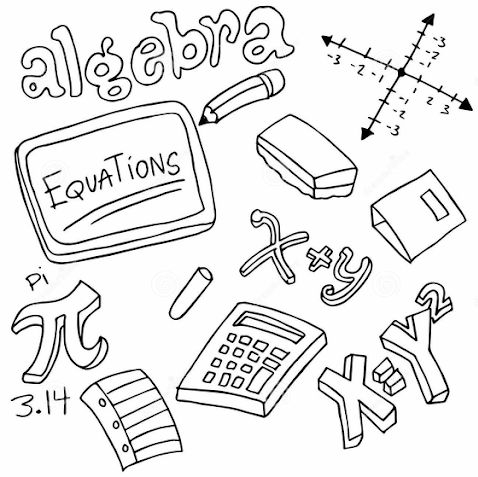# Simultaneous Linear Equations II

Welcome to Class !!

In today’s Mathematics class, We will be looking at Simultaneous Equations. We hope you enjoy the class!#### GRAPHICAL METHOD OF SOLVING SIMULTANEOUS EQUATION

Expressions in x written as ax+b where a and b are constants (which can be any number) are known as linear expression. Thus we can draw a graph representing the above expression by equating it to y. To draw a linear graph we select suitable values of x so as to calculate the values of the corresponding y. hence to draw a simultaneous equation, we make y the subject in each of the equation. Then find the values of the corresponding y with the selected suitable values of x.

Steps   in using the graphical method

1. Make y the subject in each equation.
2. Draw a table of values for each of the linear equations; taking a range of values.
3. On a graph paper label the x-axis and y-axis according to the table drawn in step 2(two) above.
4. Plot these values and join the points for each of the table of values.
5. Take note of the point of intersection of the two lines. At this point trace it to both y and x-axes. The values are the only pair of values that satisfy both simultaneous equations.

Example:

Solve graphically the simultaneous equation below

X + y =6 ; 3x –y = 12.

Solution:

1. From eq (1)  y= 6- x

From eq (2) y= 3x+2

1. Draw the table of values of the equations taking a range of values i.e.

Table for y = 6-x

 X -1 0 1 2 3 y 7 6 5 4 3

Guiding equation: Y=6-x

• when x= -1, Y =6-(-1), Y= 6 +1, Y=7
• when x=0, Y= 6-0, Y= 6
• when x=1, Y= 6-1, Y= 5
• when x=2, Y= 6 -2, Y= 4
• when x=3, Y=6-3, Y= 3

Table for Y=3x+2

Guiding equation: Y=3x+2

 X -1 0 1 2 3 y -1 2 5 8 11

• when x=-1, Y=3(-1) + 2; Y= -3+2; Y= -1
• when x= 0, Y= 3(0) + 2; Y= 0+2 = 2
• when x=1, Y=5
• when x=2, Y=8
• when x=3, Y=3(3) +2; Y=9+2=11

EVALUATION

1. What is a linear equation?
2. Which of these equations are linear? A. a+b B.a2 +b =12 C. x-1 = 2
3. What is the first step in drawing graph?

Further exercises on the use of a graph to solve a simultaneous equation

when making the table of values for points to be plotted, x is called the independent variable while y is the dependent variable. The point where the variable crosses an axis is called an intercept.

Example:

Draw the graph of the given pair of the equation 2x-y=3, x+y=6 and show the point of intercept of the lines on the y-axis.

Solution:

• Make y the subject in each equation. I.e. Y=2x-3; Y=6-x
• Make a table of values for each equation with ranges of Y=2x-3
 X -1 0 1 2 3 y -5 -3 -1 1 3
• Make the table of value for Y=6-x
 X -1 0 1 2 3 y 7 6 5 4 3

From the graph, the points of intercept are -3 and -6.

EVALUATION:

Solve graphically the below simultaneous equation:

• Y-x = -4; Y+3x =12
• 8c +3d = 1; 4c+5d =9

We have come to the end of this class. We do hope you enjoyed the class?

Should you have any further question, feel free to ask in the comment section below and trust us to respond as soon as possible.

In our next class, we will be talking about Geometry. We are very much eager to meet you there.

Are you a Parent? Share your quick opinion and win free 2-month Premium Subscription

Don`t copy text!